A. Student

Math 124 Problem Set 7

1. D=-155 There are four elements: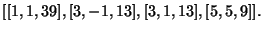By the structure theorem,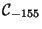is isomorphic to either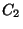xor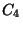. It is easy to verify that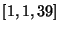is the identity. From this we find that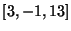has order 4, so it must be that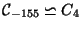.
D=-231 There are twelve elements: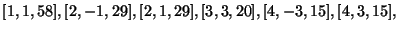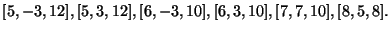Therefore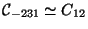orx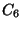. The identity is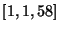. Both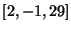and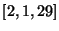have order 6, which is impossible in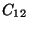, so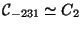x.
D=-660 There are eight elements: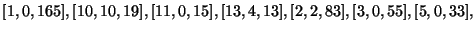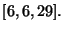The first element is the identity, and all others have order 2. Therefore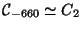xx.
D=-12104 There are forty-eight elements: (listed in an email from Professor Stein). By the structure theorem,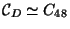,x, orx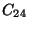. The identity element is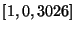, and using it we find two elements of order four: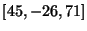and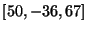, eliminating everything butx.
D=-10015 There are fifty-four elements (listed in an email from Professor Stein). Therefore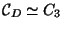x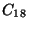or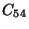. The identity is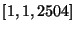; from this we find two elements with order 9: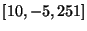and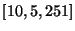. Therefore the group cannot be, sox.
2. The three graphs are on the next page, plotted in MAPLE.
3. Differentiating implicitly, the slope of the tangent at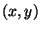is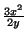. At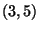, the slope is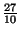, and the tangent line has equation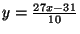. Substituting into the relation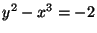, we have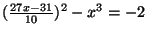, which simplifies to the polynomial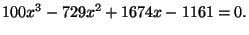This polynomial has a double root at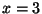, so it factors into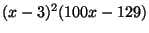, giving a rational root with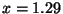. Therefore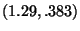is a rational solution to the original equation.